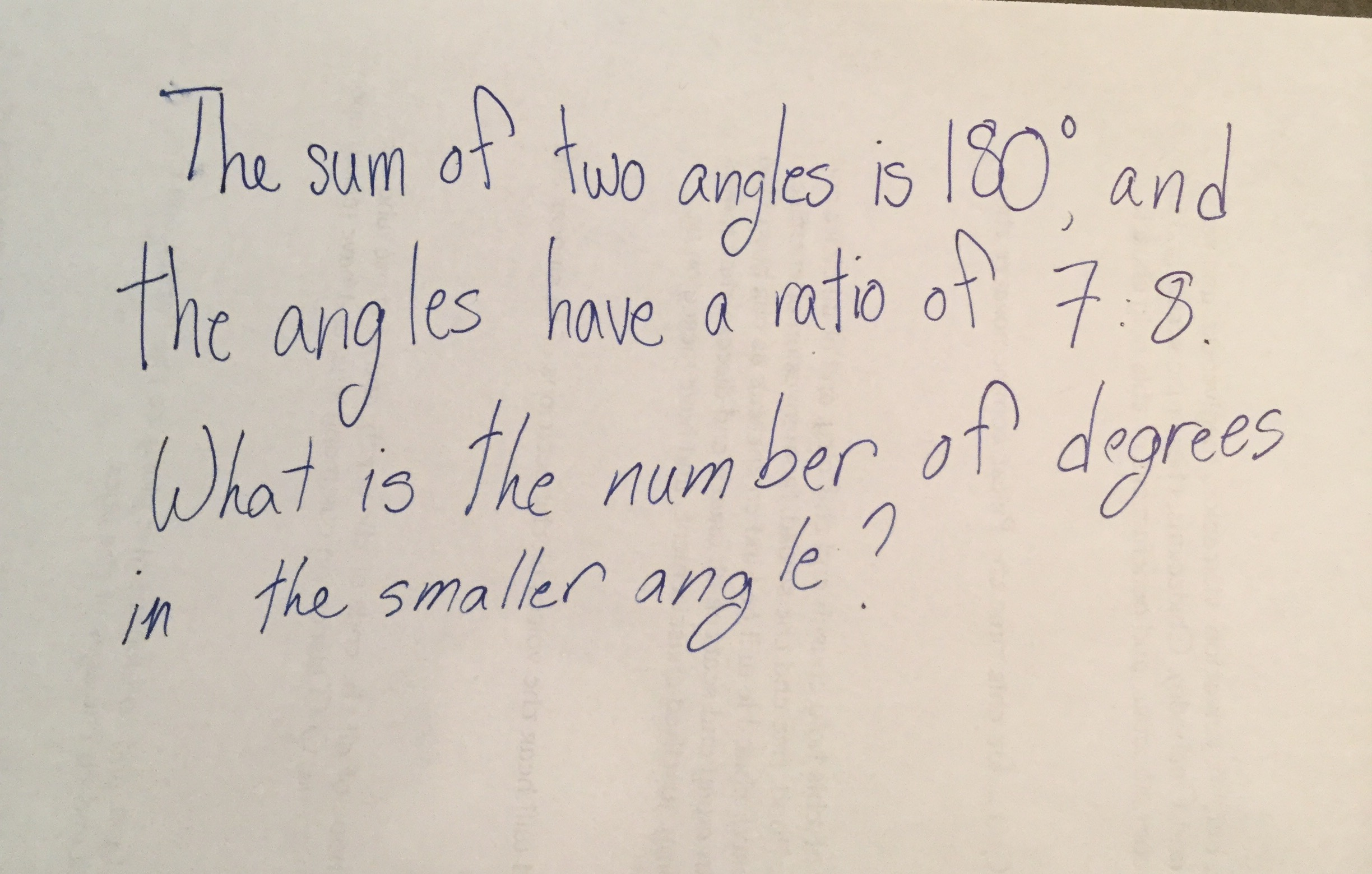D

#### How do I find an angle from a given ratio?

47 viewed last edited 4 years agoLucia Kaelin
0I have read the problem several times over and am incredibly lost. I also don't know where to start, as I have never learned this subject matter before.
I need help starting from the ground up...so absolutely everything.Vivekanand Vellanki
4
Let me re-phrase this question: a total of 180 cheese slices are divided among Leia and Luke such that for every 7 slices that Leia gets, Luke gets 8. How many slices does Leia get? Lets verify that the questions are the same: # slices that Leia gets / # slices that Luke gets = 7/8 (that is the ratio of the angles) # slices Leia gets + # slices that Luke gets = 180 (this is the sum of the two angles) We know that for every 7 slices that Leia gets, Luke gets 8 slices. So, out of a total of 15 slices, Leia gets 7 and Luke gets 8. We need to find out how many slices Leia gets among the 180 slices. To do that, we need to figure out how many of such bunches of 15 slices are present in 180 slices. Lets call a bunch of 15 slices, a pack of slices. How many such packs exist in 180 slices? That would be total number of slices divided by number of slices in a pack = 180 / 15 = 12. In each pack, Leia gets 7 slices and Luke gets 8 slices. So, in the 12 packs, Leia gets 12*7 = 84 slices. To keep it simple, I have tried doing this without using any Mathematics concepts.Lucia Kaelin
0
Thanks this help alot!Mahesh Godavarti
0
Let the smaller angle be x \text{ and the larger angle be } y . Since the ratio is 7: 8, it means that \frac{x}{y} = \frac{7}{8} . Since the sum of the angle is 180^\circ , we also have x + y = 180^\circ . Now, we have a system of equations with two variables that we will solve using the method of substitution. The first equation is x - \frac{7}{8}y = 0 . We get the first equation by multiplying both sides of \frac{x}{y} = \frac{7}{8} by y . Now, substitute the value of x in the second equation to get \frac{7}{8} y + y = 180^\circ \implies \frac{15}{8} y = 180^\circ \implies y = \frac{8}{15} 180^\circ \implies y = 96^\circ . Therefore, the smaller angle is x = \frac{7}{8} 96^\circ = 84^\circ . If you don't understand any step here, you can simply add your comment on which particular step was difficult to follow and a more detailed explanation will be provided.Lucia Kaelin
0
Thanks very much! I am a little confused as to how the smaller angle is 96 degrees, seeing as the total angle only adds up to 180. Again, thanks.Mahesh Godavarti
1
You are absolutely right Lucia! You caught a mistake. So, I had to go and fix it.